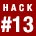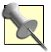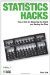# Hack 13. Use One Variable to Predict AnotherSimple linear regression is a powerful tool for measuring something you cannot see or for predicting the outcome of events that have not happened yet. With some help from our special friend statistics, you can make a precise guess of how someone will score on one variable by looking at performance on another.

Many professionals, both in and outside of the social sciences, often need to predict how a person will perform on some task or score on some variable, but they cannot measure the critical variable directly. This is a common need when making admission decisions into college, for example. Admissions officers want to predict college performance (perhaps grade point average or years until completion). However, because the prospective student has not actually gone to college yet, admissions officers must use whatever information they can get now to guess what the future holds.

Schools often use scores on standardized college admissions tests as an indicator of future performance. Let's imagine that a small college decides to use scores on the American College Test (ACT) as a predictor of college grade point average (GPA) at the end of students' first years. The admissions office goes back through a few years of records and gathers the ACT scores and freshman GPAs for a couple hundred students. They discover, to their delight, that there is a moderate relationship between these two variables: a correlation coefficient of .55.

Correlation coefficients are a measure of the strength of linear relationships between two variables [Hack #11], and .55 indicates a fairly large relationship. This is good news because the existence of a relationship between the two makes ACT scores a good candidate as a predictor to guess GPA.

Simple linear regression is the procedure that produces all the values we need to cook up the magic formula that will predict the future. This procedure produces a regression line that we can graph to determine what the future holds [Hack #12], but once we have the formula, we don't actually need to do any graphing to make our guesses.

#### Cooking Up the Equation

First, examine the recipe for creating the formula (see the "Regression Formula Recipe" sidebar), and then we'll see how to use it with real data. You can clip this recipe out and keep it in the kitchen drawer.

## Regression Formula Recipe

Ingredients

2 samples of data from correlated variables:

1 criterion variable (the one you want to predict)

1 predictor variable (the one you will predict with)

1 correlation coefficient of the relationship between the 2 variables

2 sample means

2 sample standard deviations

Container

An empty equation shaped like this:

Directions

Calculate the weight by which you will multiply your predictor variable:

Calculate the constant:

Fill the regression equation with the weight and constant you just prepared.

Serves

Anyone interested in guessing what would happen if....

The regression recipe calls for two other ingredients, means and standard deviations for both variables. Here are those statistics for our example:

VariableMeanStandard deviation
ACT scores20.102.38
GPA2.98.68You can review means and standard deviations in "Describe the World Using Just Two Numbers" [Hack #2].

The admissions office built a regression equation from this information. Consequently, as each applicant's letter came into the admissions office, an officer could enter the student's ACT score into the regression formula and predict his GPA. Let's figure out the parts of the regression equation in this example:

By placing all this information into the regression equation format, we get this formula for predicting freshman GPA using ACT scores:Notice that the constant in this case is a negative number. That's OK.

#### Predicting Scores

In our college admissions example, imagine two letters arrive. One applicant, Melissa, has an ACT score of 26. The other applicantlet's call him Brucehas an ACT score of 14.

Using the regression equation we have built, there would be two different predictions for these folks' eventual grade point averages:

For Melissa

• Predicted GPA = -.24 + (26x.16)

• Predicted GPA = -.24 + 4.16

• Predicted GPA = 3.90

For Bruce

• Predicted GPA = -.24 + (14x.16)

• Predicted GPA = -.24 + 2.24

• Predicted GPA = 2.00

I hope, for Bruce's sake, there is more than one spot available.The two variables in this example, ACT scores and GPA, are on different scales, with ACT scores typically running between 1 and 36 and GPA ranging from 0 to 4.0. Part of the magic of correlational analyses is that the variables can be on all sorts of different scales and it doesn't matter. The predicted outcome somehow knows to be on the scale of the criterion variable. Kind of spooky, huh?

#### Why It Works

When two variables correlate with each other, there is overlap in the information they provide. It is as if they share information. Statisticians sometimes use correlational information to talk about variables sharing variance.

If some of the variance in one variable is accounted for by the variance in another variable, it makes sense that smart mathematicians can use one correlated variable to estimate the amount of variance from the mean (or distance from the mean) on another variable. They would have to use numbers that represent the variables' means and variability, and a number that represents the amount of overlap in information. Our regression equation uses all that information by including means, standard deviations, and the correlation coefficient.

#### Where Else It Works

Regression is helpful in answering research questions beyond making predictions. Sometimes, scientists just want to understand a variable and how it operates or how it is distributed in a population. They can do this by looking at how that variable is related to another variable that they know more about.Statisticians call simple linear regression simple not because it is easy, but because it uses only one predictor variable. It is simple as compared to complex. Real-life predictions like those in our example usually use many predictors, not just one. The method of predicting a criterion variable using more than one predictor is called multiple regression [Hack #14].

#### Where It Doesn't Work

There will be error in predictions under three circumstances. First, if the correlation is less than perfect between two variables, the prediction will not be perfectly accurate. Since there are almost never really large relationships between predictors and criteria, let alone perfect 1.0 correlations, real-world applications of regression make lots of mistakes. In the presence of any correlation at all, though, the prediction is more accurate than blind guessing. You can determine the size of your errors with the standard error of estimate [Hack #18].

Second, linear regression assumes that the relationship is linear. This is discussed in "Graph Relationships" [Hack #12] in greater detail, but if the strength of the relationship varies at different points along the range of scores, the regression prediction will make large errors in some cases.

Finally, if the data collected to first establish the values used in the regression equation are not representative of future data, results will be in error. For example, in our college admissions example, if an applicant presents with an ACT score of 36, the predicted GPA is 5.52. This is an impossible value that does not even fit on the GPA scale, which maxes out at 4.0. Because the past data that was used to establish the prediction formula included few or no ACT scores of 36, the equation was not equipped to deal with such a high score.Statistics Hacks: Tips & Tools for Measuring the World and Beating the Odds
ISBN: 0596101643
EAN: 2147483647
Year: 2004
Pages: 114
Authors: Bruce Frey

Similar book on Amazon